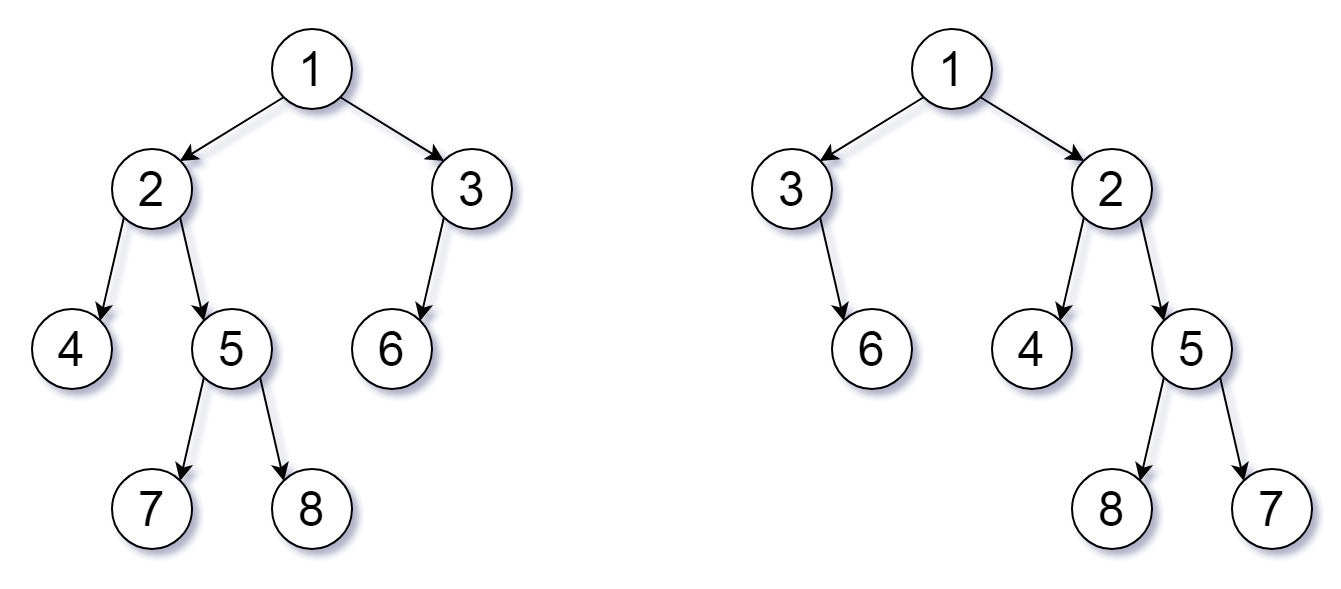# 951. Flip Equivalent Binary Trees

Medium
For a binary tree T, we can define a flip operation as follows: choose any node, and swap the left and right child subtrees.
A binary tree X is flip equivalent to a binary tree Y if and only if we can make X equal to Y after some number of flip operations.
Given the roots of two binary trees `root1` and `root2`, return `true` if the two trees are flip equivalent or `false` otherwise.
Example 1:Input: root1 = [1,2,3,4,5,6,null,null,null,7,8], root2 = [1,3,2,null,6,4,5,null,null,null,null,8,7]
Output: true
Explanation: We flipped at nodes with values 1, 3, and 5.
Example 2:
Input: root1 = [], root2 = []
Output: true
Example 3:
Input: root1 = [], root2 = 
Output: false
Constraints:
• The number of nodes in each tree is in the range `[0, 100]`.
• Each tree will have unique node values in the range `[0, 99]`.

### 解題

Runtime: 0 ms, faster than 100%
Memory Usage: 2.4 MB, less than 77.78%
/**
* Definition for a binary tree node.
* type TreeNode struct {
* Val int
* Left *TreeNode
* Right *TreeNode
* }
*/
func flipEquiv(root1 *TreeNode, root2 *TreeNode) bool {
if root1 == nil && root2 == nil { // 兩個都nil，相等，回傳true
return true
}
if root1 == nil || root2 == nil || root1.Val != root2.Val {
// 只有一邊nil或root值不相等，樹必然不相同，回傳false，
return false
}
return (flipEquiv(root1.Left, root2.Left) && flipEquiv(root1.Right, root2.Right)) || (flipEquiv(root1.Left, root2.Right) && flipEquiv(root1.Right, root2.Left))
// 不用交換樹就相同 || 交換後樹變相同
}